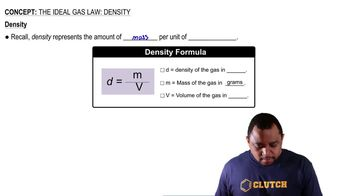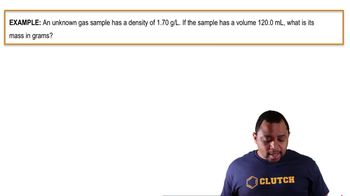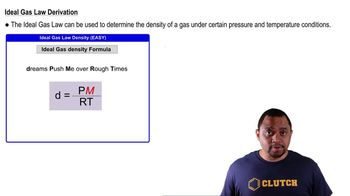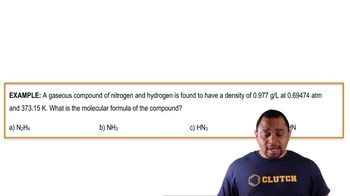Start typing, then use the up and down arrows to select an option from the list.# The Ideal Gas Law: Density Example 2

Jules Bruno
139views
here we're told that a gaseous compound of nitrogen and hydrogen is found to have a density of 0.977 g per leader at 0.69474 atmospheres and 3 73.15 kelvin. What is the molecular formula of the compound? We'll realize here in this question they're giving us density. They're giving us pressure and they're giving us temperature. With this information, I could find the more mass of this unknown gas. So here we're going to say that dreams push me over rough times and we're going to say that we have density. We have pressure, we have temperature. And we always know what our is. We just need toe isolate our Mueller Mass. So multiply both sides by rt. So are our tee times d equals p times Mueller Mass divide both sides by pressure. So Mueller mass equals d r t overpay. We'll take the information given to us. Our density is 0.977 g per leader. Our is our gas constant temperatures already in Kelvin and pressure is already in atmospheres. Doing this will see that we isolate So Adam atmospheres air gone. Kelvin's air gone leaders are gone, we'll have grams per mole. So we plug this into our formula. We're going to get here as our mass 06 g per mole. So here's our Moeller Mass. All we do now is we look at the different compounds and we would just calculate their Mueller masses and see which one comes closest to this 43.6 g per mole. Now, if you did this correctly, you would see that the answer would have to be Option C. It's the one with the mass closest to our answer. It has a Mueller Mass. Approximately equal to 43.38 g per mole. So option C would be the answer for this particular question. So realize they're giving us density, pressure and temperature. That means we can use the density form of the ideal gas law to help us find Moeller Mass and then use that information to compare to the molar masses off all these gasses00:4301:2101:0301:1902:42